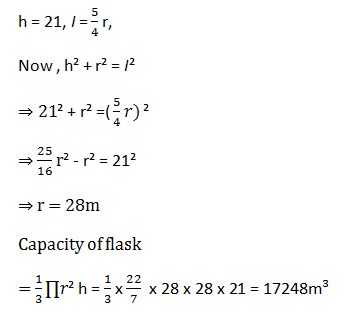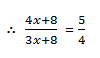# Data Sufficiency Test for IPPB Scale IDirections (1-5): Each of the questions given below consists of a question and two statements numbered I and II given below it. You have to decide whether the data provided in the statements are sufficient to answer the questions. Read both the statements and give answer

1) if the data in statement I alone are sufficient to answer the question, while the data in statement II alone are not sufficient to answer the question.
2)if the data in statement II alone are sufficient to answer the question, while the data in statement I alone are not sufficient to answer the question.
3) if the data either in statement I alone or in statement II alone are sufficient to answer the question.
4) if the data in both the statements I and II together are not sufficient to answer the question.
5) if the data in both the statements I and II together are necessary to answer the question.

#### Ques 1.

What is the capacity of a conical flask whose height is 21m?
I. Curved surface area of the flask is 3080m².

#### Ques 2.

The age of Rahul and Deepak are in the ratio of 8:9. What is the age of Rahul's wife, if she if eight years younger than Deepak?
I. The age of Rahul the Trisha are in the ratio of 4:3.
II. After 8 years the ratio of the ages of Rahul and Trisha will be 5:4.

#### Ques 3.

The speed of which of the following trains is slower?
I. Train X of length 400m crosses another Train Y of length 300m in 20 seconds moving towards each other.
II. Train X crosses a platform of length 200 in 30 seconds while Train Y crosses a pole in 20 seconds.

#### Ques 4.

Sumitra and Sushil started a business by investing the capitals in the ratio of 5:6 What is the amount of profit earned by Shilpa?
I. Shilpa joined them after 6 months investing the same amount as Sushil. After one year profit was earned, 43% of which was equal Rs. 25585.
II. Shilpa joined them after 8 months investing the same amount as Sumitra.

#### Ques 5.

What will be the cost of 2 beds,  4 chairs and 3 tables?
I. The cost of five chairs and three tables is Rs. 6220. The cost of one chair is Rs. 420 less than the cost of one table.
II. The cost of one bed is equal to the cost of 3 chairs and 2 tables.

1. 3;
From statement I;From statement II;2. 5;
From both statement I and statement II;
Rahul = 4x; Trisha = 3x⇒ 16x + 32 = 15x + 40
∴ x = 8
Rahul's age = 32 years
∴ Deepak's age =∴ Rahul's wife 's age = 36 - 8 = 28 years

3. 5;4. 1;
From statement I;
Ratio of Sumrita, Sushil and Shilpa
= 12 x 5 : 12 x 6 : 6 x 6 = 5 : 6 : 3
Now, 43% = 25585
⇒ 100% = Rs. 59500
∴ Share of Shilpa = 3/14 x 59500 = Rs, 12750

5. 5;
From both statement I and statement II;
5C+ 3T = 6220 ....(i)
and C - T - 420 ....(ii)
Solving (i) and (ii)
5T - 2100 + 3T = 6220
8T = 8320 , IT, Rs. 1040, IC = Rs. 620
Cost of OB = 3C + 2T = 1860 + 2080 = Rs. 3940
∴ Required answer = 2B + 4C + 3T = Rs. 13480